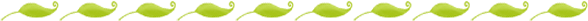# The Equal Farm

As we've been discussing plants and animals and enjoying the spring time. I've been trying to incorporate more thematic teaching into my math lessons. Here is the math lesson on the equal sign that we did today, plus the worksheet and examples that go with it as a freebie (that is, if I got google docs working correctly).

Place a large “Equal Barn” on the easel where all of the children can see it. Tell the children, “Welcome to the equal farm. At this farm, the math sign = is very important. The equal sign means the same as. Only equations that are the same on both sides can live on this farm.”

Show the children one of the examples and help them to read it in this way, “5 is the same as 4”. Then ask, “Is that true? No! 5 is not the same as 4. This equation is not the same on both sides. It cannot live on the equal farm.”

Show the children another example, “This one says, 6 is the same as 6. Is that true? Yes! This equation is the same on both sides of the equal sign. It can live on the farm.” Choose a volunteer to tape the equation to the equal barn.”

Continue with all of the example equations.

After all of the example equations have either been added to the farm or discarded, give each child a "Equal Farm" worksheet. They should cut out the equations that are equal and glue them into a "pen" in the farm. The equations that are not equal should be discarded.